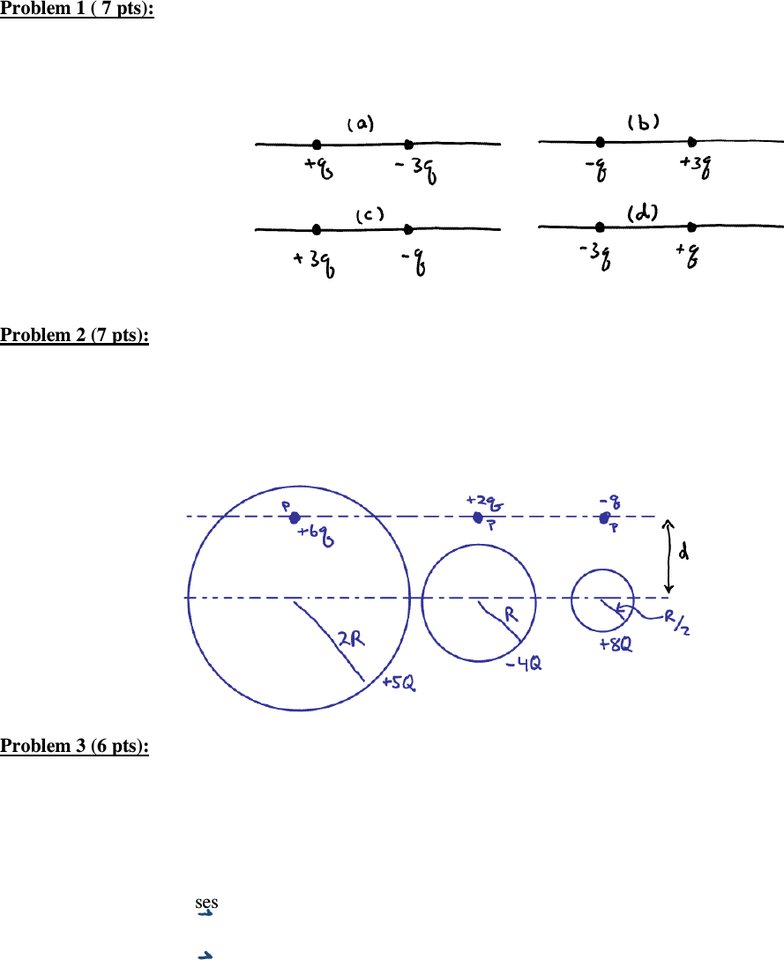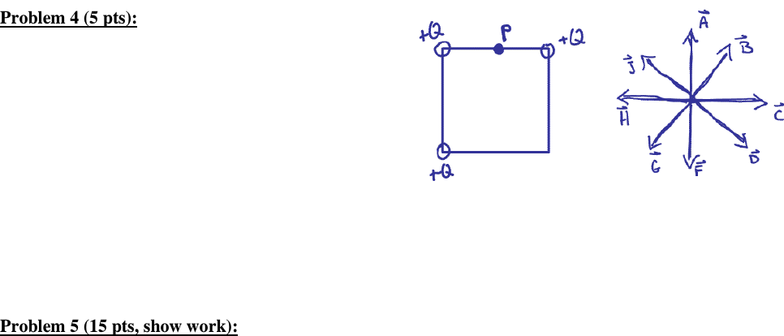Study Guides (400,000)
US (230,000)
U of R (600)
PHY (90)
PHY 114 (20)
All (20)
Midterm

# PHYS 114 University of Rochester P114 2010 Exam 1Exam

Department
Physics
Course Code
PHY 114
Professor
All
Study Guide
Midterm

This preview shows pages 1-2. to view the full 6 pages of the document.P114 University of Rochester NAME _________________________________________
S. Manly Spring 2010
Exam 1 (February 11, 2010)
the back of the page, if necessary. Show your work where indicated.
Problem 1 ( 7 pts):
The sketch below shows four situations in which charged particles are fixed in place on an axis.
In which situation(s) is there a point to the left of the particles where an electron will be in
equilibrium?
Problem 2 (7 pts):
The sketch below shows three situations involving a charged particle and a uniformly charged
spherical shell. The charges are given, and the radii of the shells are indicated. With numerals
“1”, “2” and “3”, rank the situations according to the magnitude of the force on the particle due to
the presence of the shell, greatest first. Use the same number more than once in cases where
more than one situation has the same magnitude of force.
Problem 3 (6 pts):
A closed three-dimensional surface is so constructed that, at all points on the surface, the electric
field vector points inward. Therefore, it is true that
a) the surface encloses a net positive charge.
b) the surface encloses a net negative charge.
c) the surface encloses no electric charge.
d) the area vector, dA, for each segment of the surface is necessarily parallel to the electric
field vector.
e) the area vector, dA, for each segment of the surface is necessarily perpendicular to the
electric field vector.

Only pages 1-2 are available for preview. Some parts have been intentionally blurred.P114 University of Rochester NAME _________________________________________
S. Manly Spring 2010
Problem 4 (5 pts):
A square has equal positive charges at three of its
corners, as shown in the sketch below. Circle the
vector that best shows the direction of the electric field
at point P.
Problem 5 (15 pts, show work):
A conducting ball of mass 0.2 kg is thrown straight upward from the surface of the earth with a
speed of 2 m/s. The ball carries an electric charge of 1 Coulomb and thrown upward in a big
room where there is a constant electric field of 0.5 N/C upward. To what height above the
surface of the earth does the ball rise?